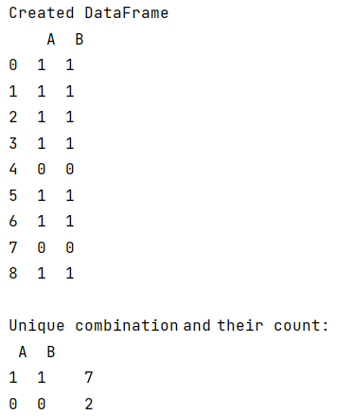# Unique combinations of values in selected columns in Pandas DataFrame and count

Given a Pandas DataFrame, we have to find the unique combinations of values in selected columns and count them.
Submitted by Pranit Sharma, on June 19, 2022

Pandas is a special tool that allows us to perform complex manipulations of data effectively and efficiently. Inside pandas, we mostly deal with a dataset in the form of DataFrame. DataFrames are 2-dimensional data structures in pandas. DataFrames consist of rows, columns, and the data.

A DataFrame column may contain similar or unique values based on the type of dataset. If a DataFrame contains two or more types of repeating values, we may need to make unique combinations of values in DataFrame and count each of these combinations and their particular values.

## Unique combinations of values in selected columns

For this purpose, we use pandas.DataFrame.value_counts() method. This method is useful when we want to count the number of each repeating value. Also, it groups all the repeating values in a column and gives their count in a separate column.

To work with pandas, we need to import pandas package first, below is the syntax:

```import pandas as pd
```

Let us understand with the help of an example,

## Python program to unique combinations of values in selected columns in Pandas DataFrame and count

```# Importing pandas package
import pandas as pd

# Creating a DataFrame
df = pd.DataFrame({
'A':[1,1,1,1,0,1,1,0,1],
'B':[1,1,1,1,0,1,1,0,1]
})

# Display DataFrame
print("Created DataFrame\n",df,"\n")

# Value count
print("Unique combination and their count:\n",df.value_counts())
```

## Output